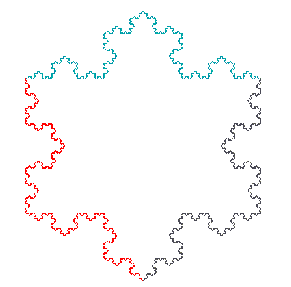Koch SnowflakeThe Koch Snowflake, described in virtually every book dealing with fractals, is a simple example of a closed figure that challenges human intuition. While it is clear that this pretty snowflake contains a finite area, not apparent is the fact that, due to its construction, its perimeter is infinite. This is only one example of a planar figure whose perimeter is indeterminate. The coastline of England, for example, is much longer when measured with a one meter ruler, than when measured with a one kilometer ruler. And longer yet when a one millimeter ruler is used. So what is the perimeter of England's coastline?

Creating a Koch Snowflake